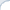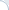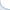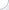This is a clarification to the previous post. Note that I am not claiming that the LSH analysis is wrong! I am saying that apparently the analysis uses a simplifying assumption. And this assumption was swept under the carpet for many years (which is quite surprising). See also a discussion in the blog of Daniel Lemire.

Yet, such approaches are quite common in CS, statistics, physics, etc... As Daniel Lemire pointed out, an analysis of regular hash tables is essentially based on a very similar assumption: that a hash function distributes elements more or less randomly. I believe that in practice this is true only to a certain a degree (and this highly depends on a hash function). Yet, we still use the analysis. So, it is probably not a big deal.

I have recently come across another (actually well-known paper), where they talk at length about the assumptions being reasonable, but make a simple math error (or at least me and my co-author think so). So, it is infinitely better to rely (on perhaps silent) assumptions, but to get the math right, then to substantiate the assumptions and totally screw the math.﻿